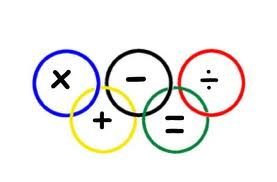# Writing mathematical symbols and numbers in words exercise

## How to complete the exercise on writing mathematical symbols and numbers in  words?

To complete the exercise write the number and mathematical symbols in words next to each number.

## Writing the mathematics and numbers exercise

 Your answer 3 + 5 = 8 three plus five is eight 12 – 2 = 10 twelve minus two is ten 37 + 6 = 28 + 4 = 69 + 7 = 218 + 83 = 625 + 195 = 1032 + 568 = 88 – 12 = 35 – 11 = 63 – 33 = 462 – 184 = 986 – 522 = 1577 – 913 =

##Lessons and exercises that are related

To view any of the lessons below click on link.

## Print the exercises

To print the exercise on matching the numbers and words right click on a white space and choose print. You can click on the printer icon just below and to the right of the contact us menu button at the top of the page or copy and paste the part of the exercise you want onto a word document and then print onto some paper.

## Dictionary and how to use dictionaries

Click on the following link for the Online English dictionary - English lesson